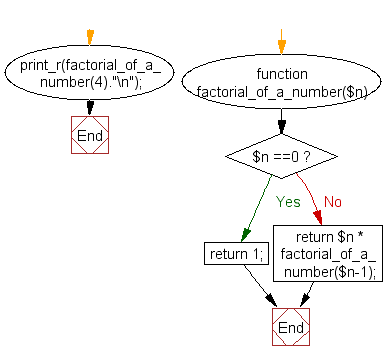﻿ PHP Function Exercise: Create a function to calculate the factorial of a positive number - w3resource# PHP function Exercises: Create a function to calculate the factorial of positive a number

## PHP function: Exercise-1 with Solution

Write a function to calculate the factorial of a number (a non-negative integer). The function accepts the number as an argument.

Pictorial Presentation:Sample Solution:

PHP Code:

``````<?php
function factorial_of_a_number(\$n)
{
if(\$n ==0)
{
return 1;
}
else
{
return \$n * factorial_of_a_number(\$n-1);
}
}
print_r(factorial_of_a_number(4)."\n");
?>
```
```

Sample Output:

```24
```

Flowchart :PHP Code Editor:

Have another way to solve this solution? Contribute your code (and comments) through Disqus.

What is the difficulty level of this exercise?

Test your Programming skills with w3resource's quiz.

﻿

## PHP: Tips of the Day

How do I strip all spaces out of a string in PHP?

Do you just mean spaces or all whitespace?

For just spaces, use str_replace:

`\$string = str_replace(' ', '', \$string);`

For all whitespace (including tabs and line ends), use preg_replace:

`\$string = preg_replace('/\s+/', '', \$string);`

Ref : https://bit.ly/2Cnb3QZ